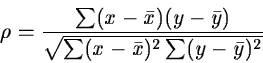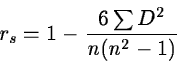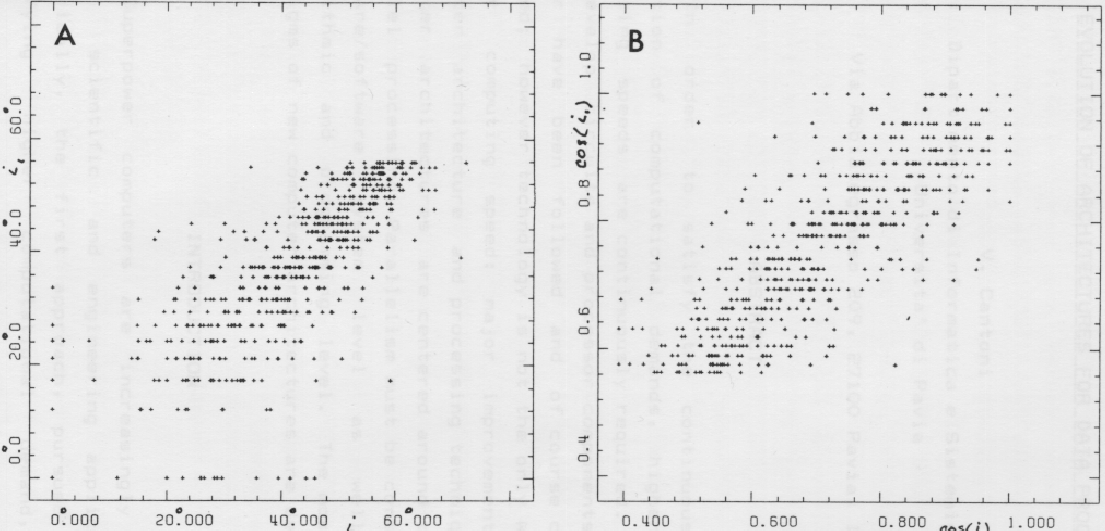Next: Statistical Tests Up: Analysis of Results Previous: Analysis of Results

Regression Analysis

The degree of correlation between two parameters can visually be estimated by plotting them. Numerically this is expressed by the correlation coefficient :(2.32)

for the two variables x and y. This expression assumes that the quantities have normal distribution. When the distribution is unknown, the correlation can be given by the Spearman rank correlation coefficient :(2.33)

where n is the number of pairs and D is the difference of the rank between x and y in a pair.

When the correlation coefficient indicates a significant correlation, the functional relation is given by the regression line which is computed by least squares methods for normal distributions or maximum likelihood procedures (see Section 2.1.3). If non-linear relations are expected, the variables are transformed into a linear domain by means of standard mathematical functions. Such transformation may also be used to make the variance of a variable homogenous.In Figure 2.13, a correlation between two different measures of the inclination angle i of galaxies is shown. Since the angle is obtained from the axial ratio, a cosine transformation is used to achieve a homogenous variance. Unfortunately, it is not always possible to obtain both a linear relation and homogeneity of variance with the same transformation.

All methods assume that the single data points are unbiased and uncorrelated. Great care must be taken to assure that this is fulfilled. If this is not the case, significant systematic errors may be introduced in the estimates. A standard example is magnitude limited samples which may be affected by the Malmquist bias.Next: Statistical Tests Up: Analysis of Results Previous: Analysis of Results
Petra Nass
1999-06-15